Anzeige

# Electricity

3. Sep 2015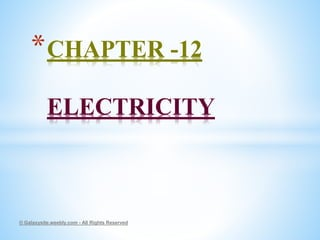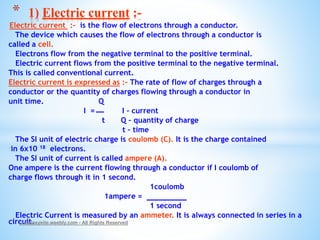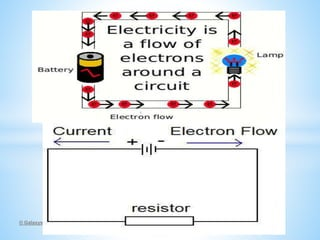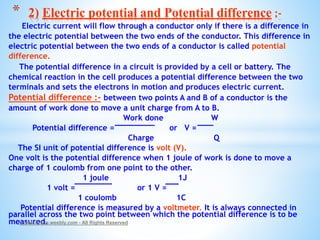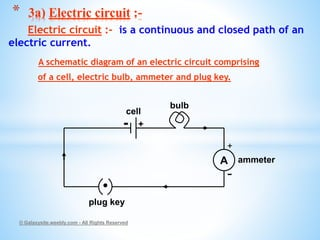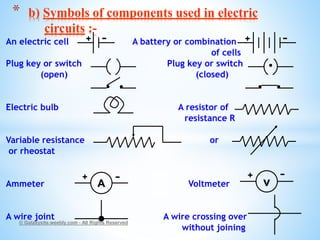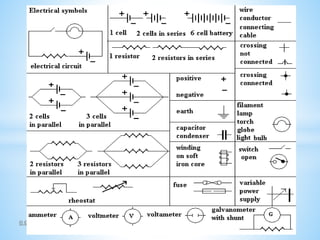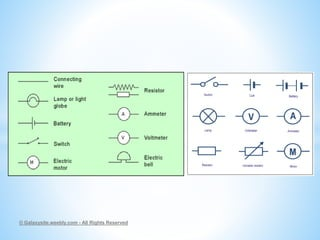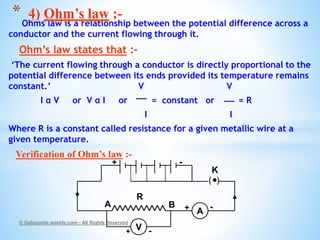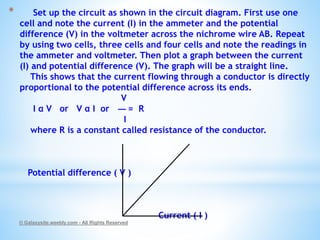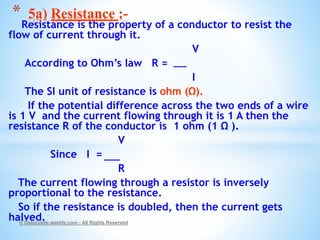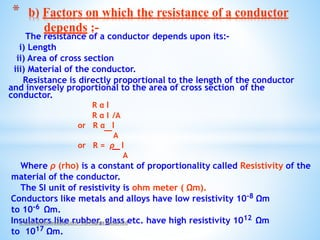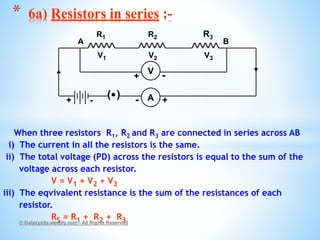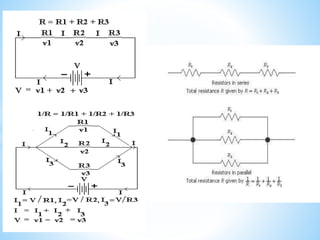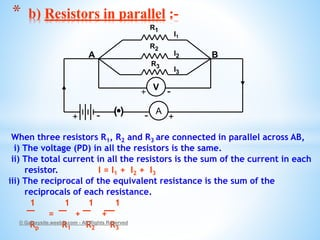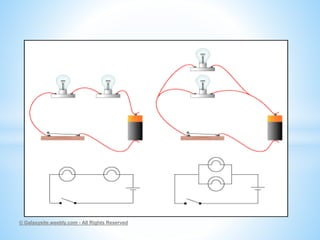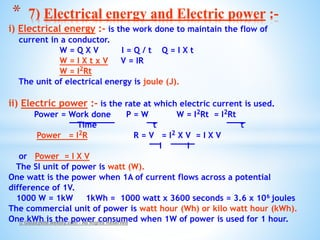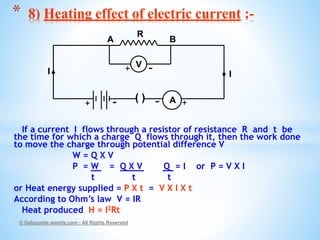1 von 18
Anzeige

### Electricity

2. Electric current :- is the flow of electrons through a conductor. The device which causes the flow of electrons through a conductor is called a cell. Electrons flow from the negative terminal to the positive terminal. Electric current flows from the positive terminal to the negative terminal. This is called conventional current. Electric current is expressed as :- The rate of flow of charges through a conductor or the quantity of charges flowing through a conductor in unit time. Q I = I – current t Q – quantity of charge t – time The SI unit of electric charge is coulomb (C). It is the charge contained in 6x10 18 electrons. The SI unit of current is called ampere (A). One ampere is the current flowing through a conductor if I coulomb of charge flows through it in 1 second. 1coulomb 1ampere = 1 second Electric Current is measured by an ammeter. It is always connected in series in a circuit.© Galaxysite.weebly.com - All Rights Reserved * 1) Electric current :-
4. Electric current will flow through a conductor only if there is a difference in the electric potential between the two ends of the conductor. This difference in electric potential between the two ends of a conductor is called potential difference. The potential difference in a circuit is provided by a cell or battery. The chemical reaction in the cell produces a potential difference between the two terminals and sets the electrons in motion and produces electric current. Potential difference :- between two points A and B of a conductor is the amount of work done to move a unit charge from A to B. Work done W Potential difference = or V = Charge Q The SI unit of potential difference is volt (V). One volt is the potential difference when 1 joule of work is done to move a charge of 1 coulomb from one point to the other. 1 joule 1J 1 volt = or 1 V = 1 coulomb 1C Potential difference is measured by a voltmeter. It is always connected in parallel across the two point between which the potential difference is to be measured.© Galaxysite.weebly.com - All Rights Reserved * 2) Electric potential and Potential difference :-
5. Electric circuit :- is a continuous and closed path of an electric current. A schematic diagram of an electric circuit comprising of a cell, electric bulb, ammeter and plug key. © Galaxysite.weebly.com - All Rights Reserved * 3a) Electric circuit :- A + - +- bulb cell ammeter plug key
6. An electric cell A battery or combination of cells Plug key or switch Plug key or switch (open) (closed) Electric bulb A resistor of resistance R Variable resistance or or rheostat Ammeter Voltmeter A wire joint A wire crossing over without joining © Galaxysite.weebly.com - All Rights Reserved * b) Symbols of components used in electric circuits :- A v+ - -+ + - + -